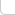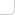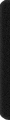# 某島

… : "…アッカリ～ン . .. . " .. .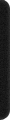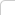April 12, 2013

## Problem A. Greg and Array

。。略）

### Analysis:

。。其實這題不需要使用線段樹或是樹狀數組。。。
。。。對這類各種操作。。一次詢問的題總有更直接的處理方法。。

。。參見咖啡樞紐。。。

## Problem C. Greg and Friends

### Brief description:

。。n 個質量分別為 1, 2 的人駕駛著一艘載重為 k 的小船渡河。
。。問把人全部送到對岸的最少往返次數。。。以及滿足最少往返次數條件下的方案數。。
( n = 50）。。

### Analysis:

… 個人認為本題十分 Nice。。。很容易想到 dp[往返次數] 然後枚舉  的人數這種五次方 DP。。。
。。。。比賽時總會有人誤認為。。返回的時候一定只搭乘1個人才能最優。。。。
。。實際上繼續觀察我們會發現。。往返這兩個過程。。並沒有本質上的差異。。。應寫成統一形式。。。。

const int N = 59;
Int f[N][N], C[N][N];
int n, k, p, q, c1, c2;

void Prep(){
swap(p, q); REP_2(a1, a2, c1+1, c2+1) f[p][a1][a2] = 0;
}

#define b (b1+b2*2)

void Roll(){
Prep(); REP_2(a1, a2, c1+1, c2+1) if (f[q][a1][a2]) REP_2(b1, b2, a1+1, a2+1) if (b &&b <= k){
f[p][c1-a1+b1][c2-a2+b2] += C[a1][b1] * C[a2][b2] * f[q][a1][a2];
}
}

int main(){

#ifndef ONLINE_JUDGE
freopen("in.txt", "r", stdin);
//freopen("out.txt", "w", stdout);
#endif

RD(n, k); k /= 50; DO(n) if (RD()==50) ++c1; else ++c2;
REP(i, n+1){C[i] = 1; REP_1(j, i) C[i][j] = C[i-1][j-1] + C[i-1][j];}

p = 0, q = 1; Prep(); f[p][c1][c2] = 1; REP(t, 2*n){

Roll();

if (f[p][c1][c2]){
printf("%d\n%d", t*2+1, int(f[p][c1][c2]));
return 0;
}

Roll();
}

printf("-1\n0");
}


## Problem D. Greg and Caves

### Brief description:

。。圖案是凸的。。上下的寬度至少是 2.。。。
。。且中間存在一個梗。（可以覆蓋其他所有行）。。
( n,m = 2000)

### Analysis:

。。大致也可以歸類到培養皿問題。。但是因為中間有一個梗而且沒有障礙。。

。。所以可以 dp 出一個方向的然後連起來。。。
。。f[][] 表示高度恰好為[]。。梗的寬度為 []。。

1 1 1 1 1 1 1
1 3 6 10 15 21 28
1 5 15 35 70 126 210
1 7 28 84 210 462 924


C(0, 0) C(1, 1) C(2, 2) C(3, 3)
C(2, 0) C(3, 1) C(4, 2) C(5, 3)
C(4, 0) C(5, 1) C(6, 2) C(7, 3)
C(6, 0) C(7, 1) C(8, 2) C(9, 3)


。。。應該可以聯繫擋板什麼的給出個比較合理的組合解釋。。。反正我是怎麼都不行。。
。。然後 s[][] 表示高度至多為 []。。然後寬度為 []。。。。
。。連起來的時候用 f[][] 和 s[][] ×起來就好。

。s[][] 可以看成是浮動窗口。。從而不會引起重複計數。。。

const int N = 2009;
Int f[N][N], s[N][N];
int n, m;

int main(){

#ifndef ONLINE_JUDGE
freopen("in.txt", "r", stdin);
//freopen("out.txt", "w", stdout);
#endif

RD(n, m);

FOR_1(i, 2, m) f[i] = 1; FOR_1(i, 2, n){Int s = 0; FOR_1(j, 2, m) f[i][j] = (s += f[i-1][j]) + f[i][j-1];}
CPY(s, f); FOR_1(i, 2, n) FOR_1(j, 2, m) s[i][j] += s[i-1][j];
Int res = 0; REP_1(i, 1) FOR_1(j, 2, m) res += s[i][j] * f[n-i+1][j] * (m-j+1);

OT(res);
}


## Problem E. Yaroslav and Points

### Brief description:

。。x 軸 上分布著 n 個點。。要求支持以下兩個操作。。

• 詢問所有範圍 [l, r] 之間的點對的距離的和。
• 移動其中一個點的坐標。

### Analysis:

… 似乎方法很多。。不過果然還是 Splay 最好弄了。。。。

/*
x
[l] [r]
*/
inline void update(){
sz = l->sz + 1 + r->sz, s = l->s + ky + r->s; // size, sum ..
ss = l->ss + (l->sz*ky-l->s) + (l->sz*r->s - r->sz*l->s) + (r->s-r->sz*ky) + r->ss; // 點對和
//      x 點和左子樹。。。     //  跨越 x 點的。。        // 和右子樹。。
}


。。對於詢問操作而言。。。是可以直接在 splay 裡面進行查找的。。但是要寫成 lower_bound upper_bound 那種著實比較麻煩。。
。。而修改操作。。也需要查找某個點的位置。。而且考慮到沒有重複結點。。寫成前驅後繼的話更不容易出錯。。

（。。xwlj 的 E 題大裸數據結構要不要這樣。。codeforces 果然也開始世風日下了么。。
http://codeforces.com/contest/295/submission/3515011